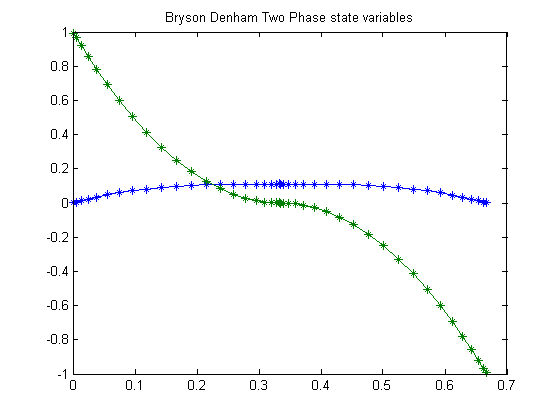20  Bryson-Denham Two Phase Problem

An example of how the input could look for PROPT.

20.1  Problem description

In this example we also take advantage of the advance knowledge that the solution reaches x1=x1max with x2=0, to introduce an event that divides the time interval into two phases. This increases the accuracy of the solution.

Reference: 

20.2  Problem setup

for n=[5 21]
toms t1 tcut
p1 = tomPhase('p1', t1, 0, tcut, n);
toms t2 tmax
p2 = tomPhase('p2', t2, tcut, tmax-tcut, n);

setPhase(p1);
tomStates x1p1 x2p1
tomControls up1
setPhase(p2);
tomStates x1p2 x2p2
tomControls up2

% Constant
x1max = 1/9;

setPhase(p1);
% Initial guess
if n==5
x01 = {tcut == 0.25
tmax == 0.5
icollocate({
x1p1 == 0
x2p1 == 1-2*t1/tcut
})
collocate(up1==0)};
else
x01 = {tcut == tcut_opt
tmax == tmax_opt
icollocate({
x1p1 == x1p1_opt
x2p1 == x2p1_opt
})
collocate(up1==up1_opt)};
end

% Box constraints
cbox1 = {0.001 <= tcut <= tmax-0.01
tmax <= 50
collocate({0 <= x1p1 <= x1max
-10 <= x2p1 <= 10})};

% Set up initial conditions in phase p1
% Initial constraints
cbnd1 = initial({x1p1 == 0; x2p1 == 1});

% ODEs and path constraints
ceq1 = collocate({
dot(x1p1) == x2p1
dot(x2p1) == up1});

% We take advantage of the fact that we've determined that a good place to
% shift between phases is when x1 reaches x1max, and that x2 must equal 0
% there (Later, we want the solver to be able to figure this out for
% itself).
% Final constraints
cbnd1 = {cbnd1
final({x1p1 == x1max; x2p1 == 0})};

% Using integral gives the integral over the phase of an expression -
% in this case 0.5 times the square of u.
% Objective
objective1 = integrate(0.5*up1.^2);

setPhase(p2);
% Initial guess
if n==5
x02 = {icollocate({
x1p2 == 0
x2p2 == 1-2*t2/tmax
})
collocate(up2==0)};
else
x02 = {icollocate({
x1p2 == x1p2_opt
x2p2 == x2p2_opt
})
collocate(up2==up2_opt)};
end

% Box constraints
cbox2 = collocate({0 <= x1p2 <= x1max
-10 <= x2p2 <= 10});

% ODEs and path constraints
ceq2 = collocate({
dot(x1p2) == x2p2
dot(x2p2) == up2});

% x2_i of p2 is already linked to x2_f of p1, but linking it to a constant
% helps convergence.
% Final conditions in phase p2.
cbnd2 = {initial(x2p2 == 0)
final(x1p2 == 0)
final(x2p2 == -1)};

objective2 = integrate(0.5*up2.^2);

final(p1,x2p1) == initial(p2,x2p2)};

20.3  Solve the problem

options = struct;
options.name = 'Bryson Denham Two Phase';
objective = objective1+objective2;
constr = {cbox1, cbnd1, ceq1, cbox2, cbnd2, ceq2, link};
solution = ezsolve(objective, constr, {x01, x02}, options);
x1p1_opt = subs(x1p1, solution);
x2p1_opt = subs(x2p1, solution);
up1_opt  = subs(up1, solution);
x1p2_opt = subs(x1p2, solution);
x2p2_opt = subs(x2p2, solution);
up2_opt  = subs(up2, solution);
tcut_opt = subs(tcut, solution);
tmax_opt = subs(tmax, solution);
Problem type appears to be: con
Starting numeric solver
===== * * * =================================================================== * * *
TOMLAB - Tomlab Optimization Inc. Development license  999001. Valid to 2011-02-05
=====================================================================================
Problem: ---  1: Bryson Denham Two Phase        f_k       3.999999993412843000
sum(|constr|)      0.000000023184469332
f(x_k) + sum(|constr|)      4.000000016597312000
f(x_0)      0.000000000000000000

Solver: snopt.  EXIT=0.  INFORM=1.
SNOPT 7.2-5 NLP code
Optimality conditions satisfied

FuncEv   39 GradEv   37 ConstrEv   37 ConJacEv   37 Iter   34 MinorIter   70
CPU time: 0.093750 sec. Elapsed time: 0.094000 sec.
Problem type appears to be: con
Starting numeric solver
===== * * * =================================================================== * * *
TOMLAB - Tomlab Optimization Inc. Development license  999001. Valid to 2011-02-05
=====================================================================================
Problem: ---  1: Bryson Denham Two Phase        f_k       3.999999993239177900
sum(|constr|)      0.000000001580905490
f(x_k) + sum(|constr|)      3.999999994820083500
f(x_0)      3.999999738758653700

Solver: snopt.  EXIT=0.  INFORM=1.
SNOPT 7.2-5 NLP code
Optimality conditions satisfied

FuncEv    4 GradEv    2 ConstrEv    2 ConJacEv    2 Iter    1 MinorIter   76
CPU time: 0.046875 sec. Elapsed time: 0.047000 sec.
end

t = subs(collocate(p1,t1),solution);
t = [t;subs(collocate(p2,t2),solution)];
x1 = subs(collocate(p1,x1p1),solution);
x1 = [x1;subs(collocate(p2,x1p2),solution)];
x2 = subs(collocate(p1,x2p1),solution);
x2 = [x2;subs(collocate(p2,x2p2),solution)];

20.4  Plot the result

figure(1)
plot(t,x1,'*-',t,x2,'*-');
title('Bryson Denham Two Phase state variables');